Thermodynamics Practice Level - 1

# Thermodynamics Practice Level - 1

Test Description

## 30 Questions MCQ Test | Thermodynamics Practice Level - 1

Thermodynamics Practice Level - 1 for Class 11 2022 is part of Class 11 preparation. The Thermodynamics Practice Level - 1 questions and answers have been prepared according to the Class 11 exam syllabus.The Thermodynamics Practice Level - 1 MCQs are made for Class 11 2022 Exam. Find important definitions, questions, notes, meanings, examples, exercises, MCQs and online tests for Thermodynamics Practice Level - 1 below.
Solutions of Thermodynamics Practice Level - 1 questions in English are available as part of our course for Class 11 & Thermodynamics Practice Level - 1 solutions in Hindi for Class 11 course. Download more important topics, notes, lectures and mock test series for Class 11 Exam by signing up for free. Attempt Thermodynamics Practice Level - 1 | 30 questions in 60 minutes | Mock test for Class 11 preparation | Free important questions MCQ to study for Class 11 Exam | Download free PDF with solutions
 1 Crore+ students have signed up on EduRev. Have you?
Thermodynamics Practice Level - 1 - Question 1

### A piston-cylinder device initially contains air at 150 kPa and 27°C. At this state, the volume is 400 litre. The mass of the piston is such that a 350 kPa pressure is required to move it. The air is now heated until its volume has doubled. Determine the total heat transferred to the air.

Detailed Solution for Thermodynamics Practice Level - 1 - Question 1

Qin – Wout = ΔU = m(u3 –u1)
m = P1V1/RT1 = 0.697 kg
u1 = u@ 300 K = 214.36 kJ/kg
u3 = u@ 1400 K = 1113.43 kJ/kg
Therefore Qin = 767 kJ.

Thermodynamics Practice Level - 1 - Question 2

### A solid whose volume does not change with temperature floats in a liquid. For two different temperatures t1 and t2 of the liquid fractions f1 and f2 of the volume of the solid remain submerged in the liquid. The coefficient of volume expansion of the liquid is equal to

Thermodynamics Practice Level - 1 - Question 3

### A cylindrical metal rod of length L0 is shaped into aring with a small gap as shown. On heating the system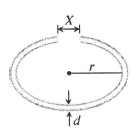Thermodynamics Practice Level - 1 - Question 4

Two holes of unequal diameters d1 and d2 (d1 > d2) are cut in a metal sheet. If the sheet is heated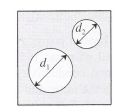Thermodynamics Practice Level - 1 - Question 5

Work done in converting 1 g of ice at - 100C intostream at 1000 C is

Thermodynamics Practice Level - 1 - Question 6

Compared to a burn due to water at 1000C, a burndue to stream at 1000C is

Thermodynamics Practice Level - 1 - Question 7

Three liquids with masses m1, m2, m3 are throughly mixed. If their specific heats are c1, c2, c3 and their temperatures T1, T2, T3, respectively, then the temperature of the mixture is

Thermodynamics Practice Level - 1 - Question 8

Two rods (one semi-circular and other straight) ofsame material and of same cross-sectional area arejoined as shown in Fig. The points A and B aremaintained at different temperatures. The ratio of theheat transferred through a cross section of a semicircularrod to the heat transferred through a crosssection of the straight rod in a given time is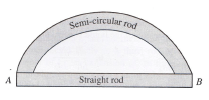Detailed Solution for Thermodynamics Practice Level - 1 - Question 8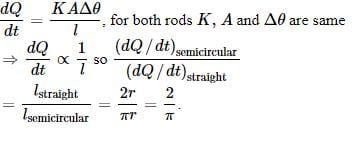Thermodynamics Practice Level - 1 - Question 9

A point source of heat of power P is placed at thecentre of a spherical shell of mean radius R. Thematerial of the shell has thermal conductivity K. Ifthe temperature difference between the outer andthe inner surface of the shell is not to exceed T, thenthe thickness of the shell should not be less than

Thermodynamics Practice Level - 1 - Question 10

Three rods of same dimensions are arranged as shownin Fig. They have thermal conductivities K1, K2 and K3.The points P and Q are maintained at different temperatures for the heat of flow at the same rate along PRQ and PQ. Which of the following options is correct?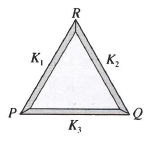Thermodynamics Practice Level - 1 - Question 11

The coefficient of thermal conductivity of copper isnine times that of steel. In the composite cylindricalbar shown in Fig., what will be the temperature atthe junction of copper and steel?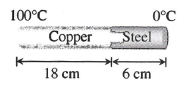Thermodynamics Practice Level - 1 - Question 12

Six identical conducting rods are joined as shown inFig. Points A and D are maintained at temperatures 2000C and 200C, respectively. The temperature of junction B will be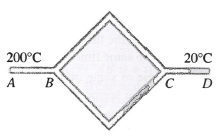Thermodynamics Practice Level - 1 - Question 13

Figure shown two air-filled bulbs connected by a U-tube partly filled with alcohol. What happens to the levels of alcohol in the limbs X and Y when an electric bulb placed midway between the bulbs is lighted?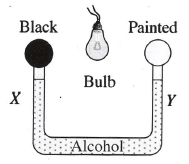Thermodynamics Practice Level - 1 - Question 14

The rectangular surface of area 8 cm × 4 cm of ablack body at a temperature of 1270C emits energy at the rate of E per second. If the length and breadth of the surface are each reduced to half of the initial value and the temperature is raised to 3270C, the rate of emission of energy will become

Thermodynamics Practice Level - 1 - Question 15

The rates of cooling of two different liquids put inexactly similar calorimeters and kept in identicalsurroundings are the same if

Thermodynamics Practice Level - 1 - Question 16

Hot water cools from 600C to 500C in the first 10 min and to 420C in the next 10 min. The temperature of the surrounding is

Thermodynamics Practice Level - 1 - Question 17

There are two thin spheres A and B of the samematerial and same thickness. They behave like black bodies. Radius of A is double that of B and both have same temperature T. When A and B are kept in a room of temperature T0 (< T), the ratio of their rates of cooling is (assume negligible heat exchange between A and B)

Thermodynamics Practice Level - 1 - Question 18

Heat is required to change 1 kg of ice at - 200C intostream. Q1 is the heat needed to warm the ice from -200C to 00C, Q2 is the heat needed to melt the ice, Q3is the heat needed to warm the water from 00C to1000C and Q4 is the heat needed to vapourize the water. Then

Thermodynamics Practice Level - 1 - Question 19

A piece of metal floats in mercury. The coeficients of volume expansion of the metal and mercury are γ1 and γ2 , respectively. If the temperatures of both mercury and the metal are increased by ΔT , the fraction of the volume of the metal submerged inmercury changes by the factor of

Thermodynamics Practice Level - 1 - Question 20

Three rods of identical cross-sectional area and madefrom the same metal form the sides of an isoscelestriangle ABC right angled at B. The points A and Bare maintained at temperatures T and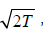,respectively, in the steady state. Assuming that only heat conduction takes place, temperature of point C is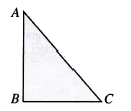Thermodynamics Practice Level - 1 - Question 21

When the temperature of a black body increases, itis observed that the wavelength corresponding to maximum energy changes from 0.26 μm to 0.13 μm.The ratio of the emissive powers of the body at therespective temperatures is

Thermodynamics Practice Level - 1 - Question 22

A box contains N molecules of a perfect gas attemperature T1 and pressure P1. The number ofmolecules in the box is doubled keeping the totalkinetic energy of the gas same as before. If the newpressure is P2 and temperature T2, then

Thermodynamics Practice Level - 1 - Question 23

The expansion of units mass of a perfect gas atconstant pressure is shown in Fig. Here.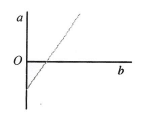Thermodynamics Practice Level - 1 - Question 24

A gas is filled in the cylinder shown in Fig. The twopistons are joined by a string. If the gas is heated,the right piston will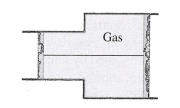Thermodynamics Practice Level - 1 - Question 25

If pressure of a gas contained in a closed vessel inincreased by 0.4% when heated by 10C, the initial temperature must be

Thermodynamics Practice Level - 1 - Question 26

Pressure versus temperature graph of an ideal gasof equal number of moles of different volumes isplotted as shown in Fig. Choose the correctalternative.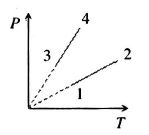Thermodynamics Practice Level - 1 - Question 27

​A gas is heated at a constant pressure. The fraction of heat supplied used for external work is

Thermodynamics Practice Level - 1 - Question 28

A monatomic gas expands at constant pressure onheating. The percentage of heat supplied thatincrease the internal energy of the gas and that isinvolved in the expansion is

Thermodynamics Practice Level - 1 - Question 29

The average degrees of freedom per molecule for a gas are 6. The gas performs 25 J of work when it expands at constant pressure. The heat absorbed y gas is

Thermodynamics Practice Level - 1 - Question 30

A and B are two isolated spheres kept in closeproximity so that they can exchange energy byradiation. The two spheres have identical physicaldimensions but the surface of A behave like aperfectly black body while the surface of B reflects 20% of all the radiations it receives. They are isolatedfrom all other sources of radiation.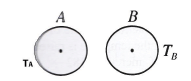Use Code STAYHOME200 and get INR 200 additional OFF Use Coupon Code
Information about Thermodynamics Practice Level - 1 Page
In this test you can find the Exam questions for Thermodynamics Practice Level - 1 solved & explained in the simplest way possible. Besides giving Questions and answers for Thermodynamics Practice Level - 1, EduRev gives you an ample number of Online tests for practice